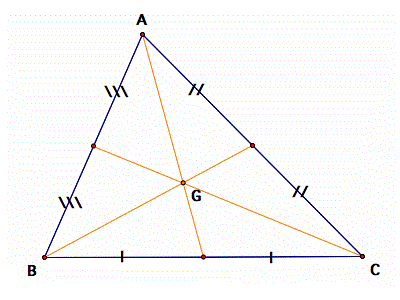# Centroid of a Triangle Calculator

#### How to Calculate Centroid of a Triangle

Enter the coordinates of the three vertices of the triangle; A(x1, y1), B(x2, y2), C(x3, y3), to calculate the centroid 'G'.

A (x1, y1)

B (x2, y2)

C (x3, y3)

The centroid of a right angle triangle is the point of intersection of three medians, drawn from the vertices of the triangle to the midpoint of the opposite sides. Centroid of a triangle calculator tool finds the mid or center point of 3 given points of a triangle on the multi-dimensional coordinate system.

## Centroid FormulaIn a traingle if the three vertices are A(x1, y1), B(x2, y2), C(x3, y3), then the centroid of a triangle 'G' can be calculated by taking the average of X and Y coordinate points of all three vertices.

Centroid of a triangle (G) = ((x1+x2+x3)/3, (y1+y2+y3)/3)

### Define Centroid

Centroid is the point of concurrence of all three medians of a triangle. It always lies inside the triangle. The centroid divides the median from vertex to mid-point of the opposite side in the ratio 2:1.

A median of a triangle is a segment from a vertex to the midpoint of the opposite side. The three medians of a triangle are concurrent. The point of concurrency, called the centroid, is inside the triangle.

#### Centroid Theorem

The centroid theorem states that the centroid of the triangle is at 2/3 of the distance from the vertex to the mid-point of the sides.### Spreading Knowledge Across the World

USA - United States of America  Canada  United Kingdom  Australia  New Zealand  South America  Brazil  Portugal  Netherland  South Africa  Ethiopia  Zambia  Singapore  Malaysia  India  China  UAE - Saudi Arabia  Qatar  Oman  Kuwait  Bahrain  Dubai  Israil  England  Scotland  Norway  Ireland  Denmark  France  Spain  Poland  and  many more....